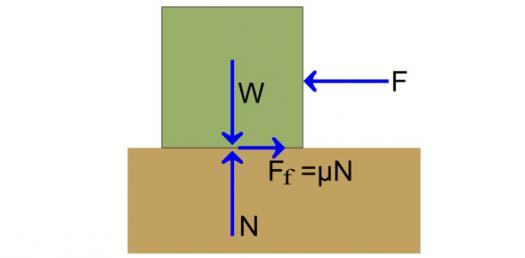# Mechanical Advantage Of The Machine

25 Questions | Total Attempts: 482SettingsComplete each question below. Each question is worth (4pts). Once completed check your score and review the test. If there is a question on the score please print out the test, mark the question of issue and turn in.

• 1.
The wheels on a bicycle have a 10 inch  radius. If the bike must travel exactly 2000 feet, how many revolutions are required? Assume that no sliding or slipping occurs between the wheel and the road.   ___________ (Round to the neareast whole number)
• 2.
If a simple machine requires an effort force that is less than the force of the load being moved, then that simple machine exhibits ______________.
• 3.
When used to pry open a can of paint, a screwdriver functions as a/an __________.
• 4.
If the pulley system shown in the figure below is lifting a 50 lb. load, what is the minimum amount of effort that must be applied to the system?  ___________
• 5.
What class of lever is shown in Figure 5?
• 6.
What is the minimum effort force that is needed to lift the 100 lb load?
• 7.
In a second-class lever, the distance from the effort to the fulcrum is ____________ than the distance from the load/resistance to the fulcrum.
• 8.
A ramp is used to raise an object 3’ from the ground. The lengths of the sides of the ramp are shown in the figure below. The mechanical advantage of the ramp is __________.
• 9.
___________ is the process of using a tap or die to produce spiral mating surfaces for the purpose of providing mechanical tension between two mating parts.
• A.

Turning

• B.

Forging

• C.

• D.

Annealing

• 10.
What class of lever is shown in Figure 5?
• 11.
If a 50 lb effort is needed to balance a 260 lb load, how far from the fulcrum should the load be located? (Answer precision = 0.0)
• 12.
1.      A 150-lb man has set up a wooden plank as a lever to move a 1000-lb rock. The rock is 3.0-ft from the fulcrum. How far does the man have to walk up the plank, from the fulcrum, before the rock starts to move? ___________v
• 13.
Tweezers are an example of which type of simple machine (be specific)? ___________
• 14.
1.       Bill is using the ramp shown to raise an  158 pound object 4 feet above the ground. How much effort will be needed to complete the task assuming no friction ________________.
• 15.
The jack pictured below has a ¾-10 screw thread and a 9-inch effort arm. It can lift an object that weighs up to 600 lbs. Calculate the mechanical advantage of this device. (nearast 0.00)
• 16.
Which of the following is a factor in calculating the mechanical advantage of a screw?
• A.

Distance between the load and the fulcrum.

• B.

• C.

• D.

Number of structural supports

• 17.
Jack and Jill are interested in bringing water up from the well. Identify the type of simple machine.
• 18.
Jack and Jill are interested in bringing water up from the well.  What is the Mechanical Advantage of the machine?
• 19.
Jack and Jill are interested in bringing water up from the well. How much effort will be needed to lift the pail of water? (Accuracy nearst 0.00)
• 20.
Jack and Jill are interested in bringing water up from the well.  Assuming the the AMA is at 2.65 and the IMA is at 3.10.  What is the efficancy of the simple machine. (accuracy nearest 0.00)
• 21.
For every 2 metres the rope is pulled through, what height does the load rise off the ground?
• 22.
Find gear ratio and find the input torque required so that the output torque is 100 ft-lb.  (Accuracy to 0.00)
• 23.
A simple gear train is composed of three gears. Gear A is the driver and has 8 teeth, gear B has 24 teeth, and gear C has 32 teeth. If the output is at C, what is the gear ratio?
• A.

3

• B.

2.5

• C.

4

• D.

6

• 24.
A simple gear train is composed of three gears. Gear A is the driver and has 8 teeth, gear B has 24 teeth, and gear C has 32 teeth. If gear A rotates at 1000 rpm, how fast is gear C rotating?
• A.

100

• B.

2500

• C.

250

• D.

25

• 25.
In a pulley system, pulley A is moving at 2000 rpm and has a diameter of 18 in. Three pulleys, B, C, and D, all of different sizes, are attached to a single output axle. Speed and torque output are changed within the system by moving the drive belt between pulleys B, C, and D. A speed of 1750 rpm is required when the drive belt is connected to pulley B. What is the diameter of pulley B?
• A.

21

• B.

18

• C.

16

• D.

24

Related TopicsBack to top